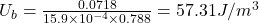## A solenoid that is 78.8 cm long has a cross-sectional area of 15.9 cm2. There are 914 turns of wire carrying a current of 8.25 A.

Question

A solenoid that is 78.8 cm long has a cross-sectional area of 15.9 cm2. There are 914 turns of wire carrying a current of 8.25 A.

(a) Calculate the energy density of the magnetic field inside the solenoid.
(b) Find the total energy in joules stored in the magnetic field there (neglect end effects).

in progress 0
7 months 2021-07-21T19:54:53+00:00 1 Answers 10 views 0

(a) Energy density will be equal to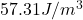(b) Total energy will be equal to 0.0718 J

Explanation:

It is given that length of solenoid l = 78.8 cm = 0.788 m

Cross sectional area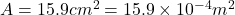Number of turns of the wire N = 914

Current in the solenoid i = 8.25 A

Inductance of the wire is equal to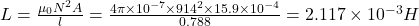(b) Total energy stored in magnetic field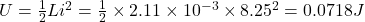(a) Energy density will be equal to